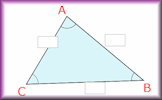# Parts of Sequences

## Find the formula that describes the part of the sequence that can be seen

##### Word 1Word 2Word 3Word 4Word 5Trophy
A

$$2n+3$$

E

$$8n+4$$

D

$$3n+1$$

H

$$n+10$$

L

$$5n-7$$

N

$$4n+3$$

V

$$6n-1$$

Y

$$7n-3$$

14, 15, 16, 17, 1813, 15, 17, 19, 213, 8, 13, 18, 23, 2823, 29, 35, 41, 4712, 20, 28, 26, 44Type the letters of the formulas for the nth terms of the parts of sequences shown above.

B

$$10-3n$$

E

$$3n+10$$

F

$$7-2n$$

H

$$7+2n$$

K

$$6-2n$$

M

$$6+2n$$

R

$$5(n-7)$$

T

$$5(7-n)$$

-15, -20, -25, -3027, 29, 31, 33, 3525, 30, 35, 40, 4516, 19, 22, 25, 2831, 34, 37, 40, 43Type the letters of the formulas for the nth terms of the parts of sequences shown above.

A

$$2^n$$

E

$$3(2^n)$$

I

$$3^n$$

L

$$2(3^n)$$

M

$$15-2^n$$

S

$$30-3^n$$

T

$$2(5-2^n)$$

U

$$2^n-7$$

6, 2, -6, -22, -543, 9, 27, 81, 24311, 7, -1, -17, -4912, 24, 48, 96, 19227, 21, 3, -51, -213Type the letters of the formulas for the nth terms of the parts of sequences shown above.

I

$$5 \times 2^n$$

U

$$(n-1)^2$$

A

$$n^3 - n^2$$

S

$$2n+n^2$$

E

$$33-n^3$$

N

$$n(n-3)$$

O

$$5n(n+1)$$

M

$$n^2-1$$

35, 48, 63, 80, 99, 12010, 20, 40, 80, 16010, 18, 28, 40, 54, 7016, 25, 36, 49, 6424, 35, 48, 63, 80, 99Type the letters of the formulas for the nth terms of the parts of sequences shown above.

A

$$\frac{n^2}{3n-1}$$

E

$$n^2-37$$

I

$$\frac{n^2}{n-1}$$

N

$$(n-2)^2$$

O

$$\frac{24}{n}$$

S

$$\frac12n(n-1)$$

T

$$\sqrt{n^3}$$

V

$$n^n$$

10, 15, 21, 28, 36, 45, 5527, 44, 63, 84, 107, 1321, 4, 27, 256, 3125, 46656-33, -28, -21, -12, -1, 1216, 25, 36, 49, 64, 81, 100Type the letters of the formulas for the nth terms of the parts of sequences shown above.

_ _ _ _ _   _ _ _ _ _   _ _ _ _ _   _ _ _ _ _   _ _ _ _ _

When you have found the five words you will see that together they make a sentence. That sentence is an instruction to do a calculation.

Enter the result of that calculation into the box below:If you have entered all five words correctly and found the result of the calculation you will be awarded a trophy.

Check

## Transum.org

This web site contains over a thousand free mathematical activities for teachers and pupils. Click here to go to the main page which links to all of the resources available.## More Activities:

Mathematicians are not the people who find Maths easy; they are the people who enjoy how mystifying, puzzling and hard it is. Are you a mathematician?

Comment recorded on the 23 September 'Starter of the Day' page by Judy, Chatsmore CHS:

"This triangle starter is excellent. I have used it with all of my ks3 and ks4 classes and they are all totally focused when counting the triangles."

Comment recorded on the 1 February 'Starter of the Day' page by Terry Shaw, Beaulieu Convent School:

"Really good site. Lots of good ideas for starters. Use it most of the time in KS3."

Each month a newsletter is published containing details of the new additions to the Transum website and a new puzzle of the month.

The newsletter is then duplicated as a podcast which is available on the major delivery networks. You can listen to the podcast while you are commuting, exercising or relaxing.

Transum breaking news is available on Twitter @Transum and if that's not enough there is also a Transum Facebook page.

#### Triangle SolverThis simple calculator will work out the lengths of the sides and the size of the angles of any triangle given thee particular pieces of information.

There are answers to this exercise but they are available in this space to teachers, tutors and parents who have logged in to their Transum subscription on this computer.

A Transum subscription unlocks the answers to the online exercises, quizzes and puzzles. It also provides the teacher with access to quality external links on each of the Transum Topic pages and the facility to add to the collection themselves.

Subscribers can manage class lists, lesson plans and assessment data in the Class Admin application and have access to reports of the Transum Trophies earned by class members.

Subscribe

## Go Maths

Learning and understanding Mathematics, at every level, requires learner engagement. Mathematics is not a spectator sport. Sometimes traditional teaching fails to actively involve students. One way to address the problem is through the use of interactive activities and this web site provides many of those. The Go Maths main page links to more activities designed for students in upper Secondary/High school.

## Teachers

If you found this activity useful don't forget to record it in your scheme of work or learning management system. The short URL, ready to be copied and pasted, is as follows:

Alternatively, if you use Google Classroom, all you have to do is click on the green icon below in order to add this activity to one of your classes.

It may be worth remembering that if Transum.org should go offline for whatever reason, there are mirror site at Transum.info that contains most of the resources that are available here on Transum.org.

When planning to use technology in your lesson always have a plan B!

Do you have any comments? It is always useful to receive feedback and helps make this free resource even more useful for those learning Mathematics anywhere in the world. Click here to enter your comments.For All: